# Outlier Exclusion Vignette

## Introduction

This vignette describes how to use Olink® Analyze to evaluate a dataset for the presence of outliers. When performing statistical analyze it is important to establish the presence of any outlier samples in the data prior to statistical analysis. There are many reasons why a sample might be an outlier, it could be due to a data entry or measurement error (i.e. labeling a control sample as a disease sample), sampling problems or unusual conditions (i.e. contamination), or natural variation. In many parametric statistics tests the mean of each group is used to determine differences between groups. Since the mean is highly sensitive to outliers, it is important to examine datasets for outliers prior to analysis as these outliers could have a large influence on the statistical results.

In Olink Analyze, there are three visualization functions that can be used to identify potential outlier samples. In this vignette, you will learn how to use olink_pca_plot(), olink_dist_plot() , and olink_qc_plot() to identify and remove outliers from a dataset.

## PCA Plot

### Overview of PCA Plot

Principal Component Analysis (PCA) is a dimensional reduction technique. PCA plots can be particularly useful to visualize variability within high dimensional data by plotting the data along the axes of greatest variation. The first two principal components make up the largest axes of variance between samples. In olink_pca_plot() samples are clustered together based on similarities in overall expression patterns of the measured proteins. These samples can be colored by any categorical variable to determine which biological factors may be contributing to global effects across the samples. PCA plots can be used to:

• Identify individual outlier samples (Figure 1A)

• Identify groups of outliers (Figure 1B)

• Identify batch effects (see Bridging Vignette)

Regardless of what the PCA is being used for, PCA plots are great tools to get an overview of the data prior to analysis and pick up on any global trends. These samples are not necessarily outliers but might be indicative of a global difference between groups.

p1<- outlier_data |> olink_pca_plot(label_samples = T, quiet = T)
p2<- group_data |> olink_pca_plot(color_g = "Site", quiet = T)
ggpubr::ggarrange(p1[], p2[], nrow = 2, labels = "AUTO")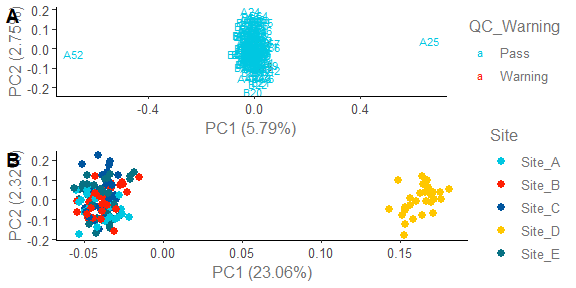Figure 1 A. PCA can be used to identify individual outlier samples as shown by samples A25 and A52. B. PCA can be used to identify difference in groups as seen by Site_D samples. This is not suggesting Site_D is an outlier, but rather that there may be a global difference between sites.

fcap <- "**Figure 1** **A.** PCA can be used to identify individual outlier samples as shown by samples A25 and A52. **B.** PCA can be used to identify difference in groups as seen by Site_D samples. This is not suggesting Site_D is an outlier, but rather that there may be a global difference between sites."

### How to use PCA plot to identify an outlier

As shown above, PCA plots can be used to identify global differences in specific samples or sets of samples. Often times these samples can be attributed to natural variations, but sometimes these samples are outliers due to technical or sample specific issues. To determine if a sample is a true outlier and should be excluded, consider the following:

• Did the sample pass QC? - Sometimes samples that are outliers and have a QC warnings may indicate sample or technical issues. For example, a buffer sample will often flag on QC and be plotted as an outlier as there are no proteins in the sample.

• How far is the sample from other samples? - Samples variability within a specific group may be larger or there may be global variables within a group. In this case it is important to consider the sample within the context of the project.

• Does the sample appear as an outlier by other plots? - If a sample is an outlier in the PCA, NPX distribution, and QC plots, that this might indicate a true outlier.

• Is the sample an outlier on all panels? - Some samples may perform better on specific samples. In the next section we will explain how to view samples by panel.

PCA plots can be generated using olink_pca_plot() and specifying the color for each sample using the color_g argument. By default the samples will be colored by QC_Warning. Prior to generating the PCA plot, the duplicate SampleIDs (often Control samples) will need to be renamed or filtered out.

OlinkAnalyze::npx_data1 |>
dplyr::filter(stringr::str_detect(SampleID, "CONTROL", negate = T)) |> # Filter duplicate SampleIDs
olink_pca_plot(color_g = "Treatment")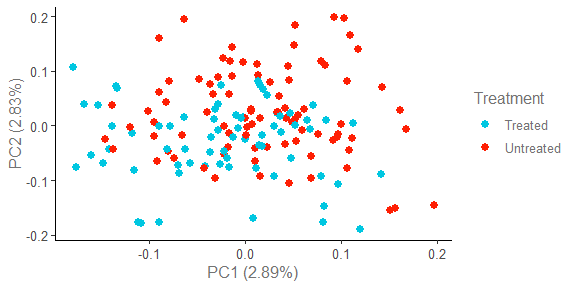In this dataset there do not appear to be any outliers in the PCA plot.

### PCA plot by panel

When multiple panels are run, there is a chance a sample may be an outlier on one panel. To get a global view of the samples per Panel, the byPanel argument can be specified.

OlinkAnalyze::npx_data2 |>
dplyr::filter(stringr::str_detect(SampleID, "CONTROL", negate = T)) |> # Filter out control SampleIDs
olink_pca_plot(byPanel = TRUE) # Specify by panel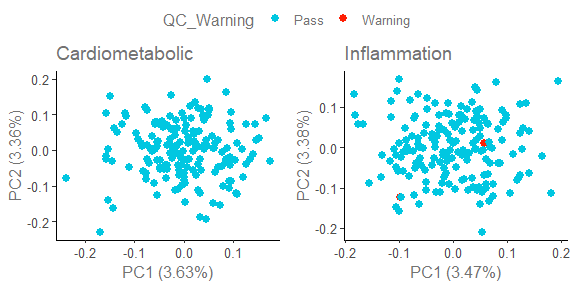The PCA plots will be saved in a list of ggplot objects and each can be viewed individually using [[n]] syntax as shown below.

pca_plots<-OlinkAnalyze::npx_data2|> # Save the PCA plot to a variable
dplyr::filter(stringr::str_detect(SampleID, "CONTROL", negate = T)) |> # Filter duplicate SampleIDs
olink_pca_plot(byPanel = TRUE, quiet = TRUE) # By panel, do not print ggarranged plot
pca_plots[] #Cardiometabolic PCA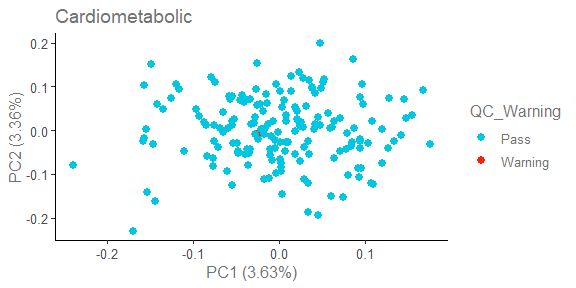pca_plots[] #Inflammation PCA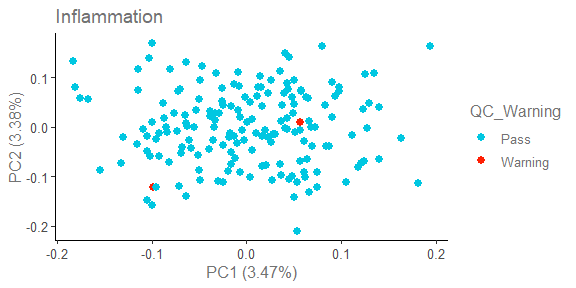The plots above show an example where there are not any clear outliers in the PCA. For the purposes of this vignette, we have also generated data where 2 samples appear as outliers.

outlier_data |>
dplyr::filter(stringr::str_detect(SampleID, "CONTROL", negate = T)) |> # Filter duplicate SampleIDs
olink_pca_plot(byPanel = TRUE)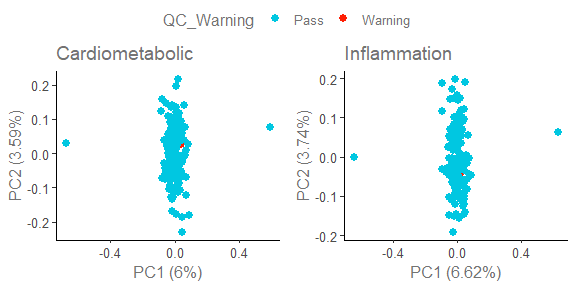However from this plot, we can not identify which two samples are outliers. In the next section we will go over how to label outliers in the plot.

### Labeling outliers

There are two ways to label the samples in the PCA plot. The first way is to use the label_samples argument. This argument will label all samples by replacing the dot with the SampleID.

outlier_data |>
dplyr::filter(stringr::str_detect(SampleID, "CONTROL", negate = T)) |> # Filter duplicate SampleIDs
olink_pca_plot(label_samples = TRUE)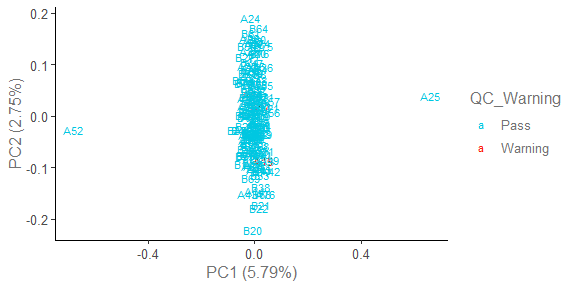Here we can see that samples A25 and A52 appear as outliers. This method can be useful when there is clear separation in samples. However it can be difficult to identify additional trends when all samples are labeled. In this case the samples must also be visually identified as opposed to programmatically extracted from the plot. For a cleaner plot with only the outliers labeled, we can use outlierDefX, outlierDefY, outlierLines, and label_outliers. These arguments will plot lines at a number of standard deviations from the mean of the plotted PC and label the samples outside of these lines. The values of outlierDefX and outlierDefY will need to be generated by the users and may require some manual adjustment to determine the correct number to highlight the outliers.

outlier_data |>
dplyr::filter(stringr::str_detect(SampleID, "CONTROL", negate = T)) |> # Filter duplicate SampleIDs
olink_pca_plot(outlierDefX = 3, outlierDefY = 3,
outlierLines = TRUE, label_outliers = TRUE)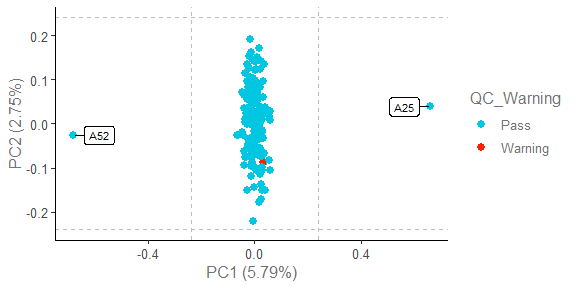To remove the lines and just keep the outliers labelled, outlierLines can be set to False.

outlier_data |>
dplyr::filter(stringr::str_detect(SampleID, "CONTROL", negate = T)) |> # Filter duplicate SampleIDs
olink_pca_plot(outlierDefX = 3, outlierDefY = 3,
outlierLines = FALSE, label_outliers = TRUE)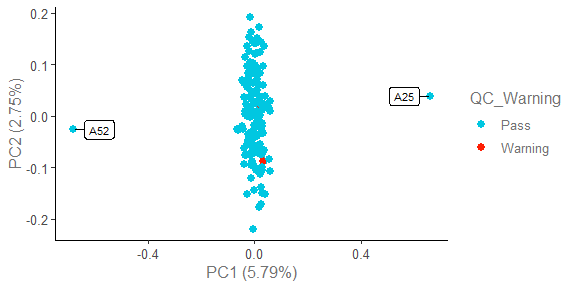Once the correct outliers have been highlighted in the graph, we can then programmatically extract the outlier SampleIDs using dplyr.

outliers_pca_labeled <- outlier_data |>
dplyr::filter(stringr::str_detect(SampleID, "CONTROL", negate = T)) |> # Filter duplicate SampleIDs
olink_pca_plot(outlierDefX = 3, outlierDefY = 3, outlierLines = FALSE,
label_outliers = TRUE, quiet = TRUE)

outliers_pca_labeled[]$data |> dplyr::filter(Outlier == TRUE) |> dplyr::select(SampleID) |> dplyr::distinct() ## SampleID ## 1 A25 ## 2 A52 ## NPX Distribution Plot ### Overview of NPX Distribution Plot NPX distribution plots generated by olink_dist_plot consist of box and whisker plots of NPX distribution for each sample. These boxplots can be used to determine if a sample has an unusually large or small distribution or if a samples NPX distribution is shifted compared to other samples in the study. When used in combination with PCA and QC plots, NPX distribution plots can give an additional dimension of data to help identify outlier samples or global trends within groups of samples. ### How to use NPX distribution plot to identify an outlier In NPX distribution plot an outlier may show one of the following characteristics: • A larger NPX distribution than other samples suggesting that the sample may contain a global difference in proteins, potentially indicating a different sample phenotype or technical error. • A smaller NPX distribution than other samples suggesting that the sample may contain less protein than other samples, often seen when running buffer samples. • A shift in NPX distribution in one or more samples suggesting a difference in overall protein concentration, a difference in sample collection methodology, or a batch effect. If we look at a subset of the outlier data from the PCA example, we can see that A25 and A52 have shifted NPX distributions, which in combination with the PCA plot, suggest that these samples may be potential outliers. If there are many samples in a project, it can be helpful to look at a subset of the samples at a time as shown below. outlier_data |> dplyr::filter(SampleID %in% c("A25", "A52", "A1", "A2", "A3", "A5", "A15", "A16", "A18", "A19", "A20"))|> olink_dist_plot()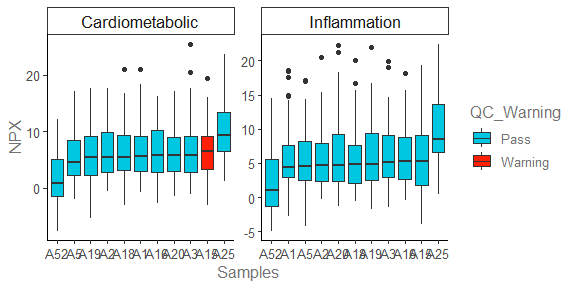NPX distribution plots can also be useful in identifying group trends, such as a difference in site D as shown in the figure below. The color of the bars can be altered using the color_g argument. group_data |> dplyr::filter(Site != "Site_E") |> # Site E filtered out so that all samples can be seen olink_dist_plot(color_g = "Site")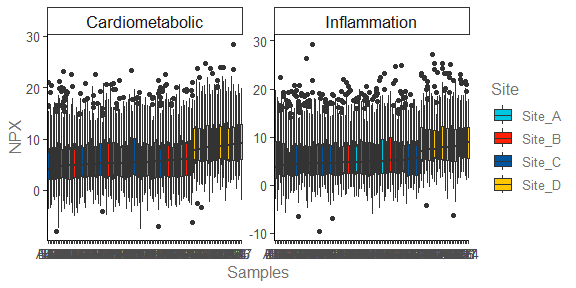In this case, the shift in NPX distribution could be biologically meaningful or could indicate a sample or technical issue. There are several biological cases where the total protein concentration in a sample may be larger in one group than another. However, in some cases the difference in protein concentration can also lead to skewed results, in which case it may be helpful to normalize the data for the changes in protein concentration by performing a median adjustment as shown below. # Calculate SampleID Median NPX median_NPX<-group_data |> dplyr::group_by(SampleID) |> dplyr::summarise(Median_NPX = median(NPX)) # Adjust by sample median adjusted_data <- group_data |> dplyr::inner_join(median_NPX, by = "SampleID")|> dplyr::mutate(NPX = NPX - Median_NPX) adjusted_data|> dplyr::filter(Site != "Site_E") |> #Filter out Site E to see all the samples olink_dist_plot(color_g = "Site")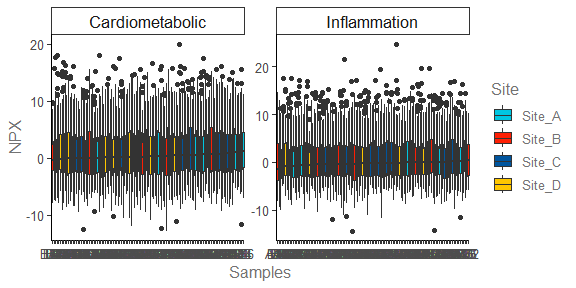## QC Plot (IQR vs Sample Median) ### Overview of QC Plot Often there are too many samples to identify outliers using olink_dist_plot(). In this case, olink_qc_plot() offers an alternative way to visualize the NPX distribution per sample. In this plot, the sample median and sample interquartile range (IQR) are plotted to visualize where the sample is centered and how much variability is within the sample. ### How to use QC plot to identify an outlier olink_qc_plot() contains similar features to olink_pca_plot() and can be used in a similar way. These samples can be colored by any categorical variable to determine which biological factors may be contributing to global effects across the samples or individual sample outlier. QC plots can be used to identify individual outlier samples or identify groups of outliers. In the QC plot an outlier may show one or more of the following characteristics: • A larger IQR than other samples suggesting that the sample may contain a global difference in proteins, potentially indicating a different sample phenotype or technical error. • A smaller IQR than other samples suggesting that the sample may contain less protein than other samples, often seen when running buffer samples. • A shift in sample median in one or more samples suggesting a difference in overall protein concentration, a difference in sample collection methodology, or a batch effect. Using the outlier data from the previous plots, we can see that sample A52 has a lower sample median and sample A25 has a higher sample median in both panels. Sample A48 has the highest IQR of all samples in the Inflammation panel. However, we can see many other samples approaching the line threshold line (default of 3 standard deviation from the mean IQR or sample median), suggesting that this sample may not be a true outlier. outlier_data |> olink_qc_plot()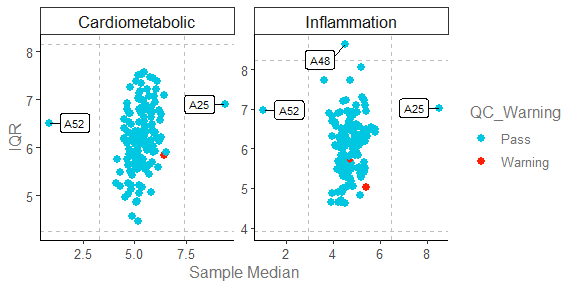With a standard deviation of 3 and assuming a normal distribution of samples, it is expected that over 99% of the data will lie within 3 standard deviations from the mean, however depending on the groups and samples within the study, there may be global shifts which will result in one or more samples outside of the 3 SD line. These lines should be used as guidelines and not strict thresholds of outliers. Similar to what was shown in the NPX distribution plot example, the QC plot can also be used to see changes in specific groups. In the example below, the samples are colored by site and Site D appears to have samples with higher sample median. group_data |> olink_qc_plot(color_g = "Site")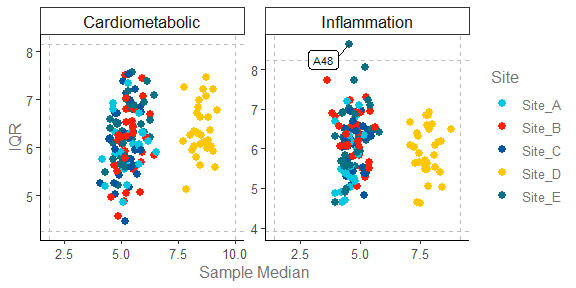### Labeling outliers To change the threshold and outliers that are labeled, we can use median_outlierDef, IQR_outlierDef, outlierLines, and label_outliers. These arguments will plot lines at a number of standard deviations from the mean of the sample median or IQR and label the samples outside of these lines. The values of median_outlierDef and IQR_outlierDef default to 3 and may require some manual adjustment to determine the correct number to highlight the outliers. outlier_data |> olink_qc_plot(median_outlierDef = 2, IQR_outlierDef = 4, outlierLines = TRUE, label_outliers = TRUE)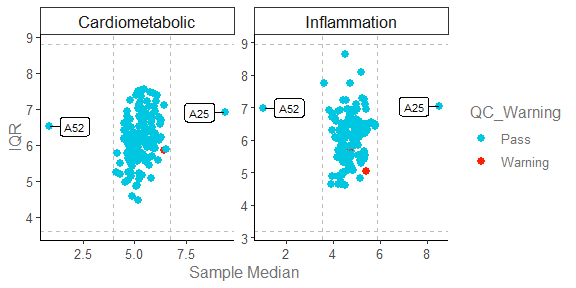To remove the lines and just keep the outliers labelled outlierLines can be set to False. outlier_data |> olink_qc_plot(median_outlierDef = 2, IQR_outlierDef = 4, outlierLines = FALSE, label_outliers = TRUE)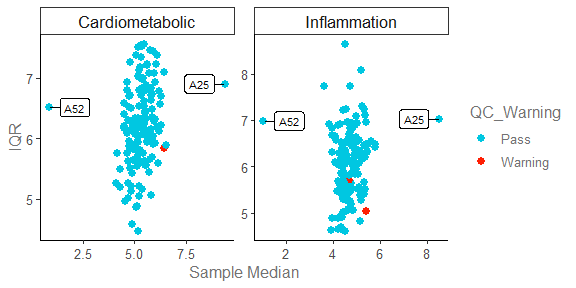Once the correct outliers have been highlighted in the graph, we can then programmatically extract the outlier SampleIDs using dplyr. outliers_qc_labeled <- outlier_data |> olink_qc_plot(median_outlierDef = 2, IQR_outlierDef = 4, outlierLines = FALSE, label_outliers = TRUE) outliers_qc_labeled$data |>
dplyr::filter(Outlier == TRUE) |>
dplyr::select(SampleID) |>
dplyr::distinct()
## # A tibble: 2 × 1
##   SampleID
##   <chr>
## 1 A25
## 2 A52

## Interpreting and Handling Outliers

### When should outliers be removed

After reviewing these plots, a list of potential outliers may be generated. Whether or not these outliers should be excluded depends on how different from the other samples the potential outliers appear and if the outliers can be traced to a biological, sample specific, or technical issue. An outlier could also be specific to a particular panel, in which case the outlier either be excluded from just the panel on which it is an outlier or excluded from all panels.

In the case of a biological issue, it may be useful to keep the potential outlier within the study as the sample has biological meaning, however additional normalization may be needed. For example, in a diabetes study, patients with severe diabetes may have higher protein in their urine as compared to control subject. In this case it could be useful to normalize based on total protein concentration or median NPX to set all values within the context of the study. Another example is in the case of multiple batches, one batch may appear to cluster differently from another batch. In this case additional normalization such as bridging is needed to bridge the studies to the same scale.